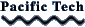## Graphing Calculator

##### for Macintosh, Windows, & iOS

Graph functions in two and three dimensions, explicit, implicit, or parametric. Graph inequalities, contour plots, density plots and vector fields. Use rectangular, polar, cylindrical, or spherical coordinates. Solve equations numerically, graphically, or symbolically.

"Graphing Calculator is one of the best examples of elegant power and clean user interface of any application I've seen." - Bruce Horn, creator of the Macintosh Finder.

Features
Two dimensions
Three Dimensions
Complex variables
Coordinate transformations
Coordinate Systems
Numeric Solutions
• Solve for zeros of any curve
• Find intersections of curves
• Identify minima and maxima
• Evaluate numeric expressions

Symbolic Methods

Animation
• Very easy to animate objects
• Slider to explore curve families

Other Features
• Graph multiple equations
• Define functions and variables
• Trigonometric and logarithmic functions
• Floor, ceiling, min, max, mod

Features only on Macintosh and Windows

## Graphing Calculator Lite

##### for Macintosh

Graphing Calculator Lite is a smaller, simpler, lower-priced version of Graphing Calculator 4.0.

Click here for the Graphing Calculator Lite features.

Graphing Calculator Lite is available only on the Mac App Store.

## Equation Calculator

##### for Macintosh

Equation Calculator is available only on the Mac App Store.

Feature comparison
Equation
Calculator
GC LiteGC 4.0GC on iOS
Symbolic computation
Simplify expressions
Evaluate derivatives
Numeric integration
Drag algebra
Complex numbers
Sliders
Animation
Create web pages
Create movies
Tables
Matrix operations
Vector operations
Text
2D Graphs
Data
Points
Functions
Inequalities
Implicit relations
Graph derivatives
Graph integrals
Parametric curves
Contour plots
Density plots
Color maps
Vectors
Vector fields
Differential equations
Coordinate transforms
3D Graphs
Data
Points
Functions
Implicit relations
Graph derivatives
Graph integrals
Surfaces of Revolution
Parametric Curves
Parametric Surfaces
Vectors
Vector fields
Differential equations
Coordinate transforms